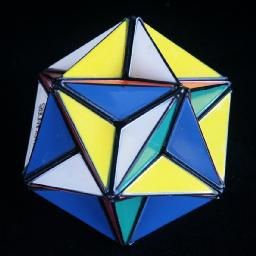# Alexander's StarThis puzzle is in the shape of a great dodecahedron, which is one of the Kepler-Poinsot polyhedra. As a puzzle it has 12 vertices which are the axes of rotation. There are 30 moving pieces which have two colours each. Each piece forms the edge between two vertices, and five such edges join at each vertex. The great dodecahedron has 12 pentagonal faces, but as these go through the interior of the solid, only part of each pentagon is visible from the outside. The visible part of a pentagon consists of a ring of five triangles. Each ring must be all of one colour, and rings on opposite sides of the puzzle must be the same colour.

This puzzle is equivalent to just the edges of the megaminx. There is however one minor difficulty with the star because only six colours are used and therefore each colour combination occurs twice.

It is not terribly difficult to solve, but this puzzle is infamously hard to twist. It was invented by Adam Alexander, a US mathematician, who patented it on 26 March 1985, US 4,506,891.

## The number of positions:

There are 30 pieces, with 2 orientations each, giving a maximum of 30!·230 positions. This limit is not reached because:
• only even permutations of pieces are possible (2)
• only and even number of flipped pieces are possible (2)
• there are 15 pairs of indistinguishable pieces (215/2 since even number of swaps)
• the orientation of the puzzle does not matter (60)

This leaves 29!·213 = 72,431,714,252,715,638,411,621,302,272,000,000 or 7.2·1034 positions. There are however 12 solved positions because there are 24 ways of arranging 5 colours around a star if you discount rotations, and two of those occur in each solution.

## Notation:

Let F, R, D be three adjacent vertices (Front, Right and Down), in other words, F, R, D are arranged clockwise at the vertices of a triangle of edges. Let U (Up) be the other vertex adjacent to both F and R, and let L (Left) be the other vertex adjacent to F and D. Clockwise 1/5 twists of a vertex are denoted by the appropriate letter, anti-clockwise 1/5 twists by F', R', L', D' or U'. In the solution below, the D vertex is usually still unsolved.

## Solution:

This puzzle is related to the Megaminx, as it is equivalent to solving only the edges of that puzzle.

1. Solve everything except the five pieces around one last vertex.
For this only two sequences are necessary. Suppose we want to place the correct piece between the F and R vertices. First find the piece on the puzzle (remember there are two possible pieces). Use any moves that don't destroy solved parts of the puzzle to place the piece somewhere on the D vertex. Then twist D and use one of the following sequences:
 DF->FR: R'D R DR->RF: F D'F'
Make sure you use the correct one, otherwise the piece is put in upside down. If a piece is upside down in position, simply use either sequence to displace it and then place it correctly. These sequences only disturb the D vertex edges and the FR edge but nothing else.
2. Position the edges of the final vertex.
Rotate D to place as many edges as possible in their correct places. You might find that the edges are in an odd permutation, for example you may have three pieces correct and two that need to be swapped. In this case you will have to swap any two identical pieces elsewhere on the puzzle and try solving it again.
In this and the next phase, the vertices adjacent to D are in clockwise order: L, F, R, b, B.
Hold the puzzle so that you can use one of the following:
 DR->DF->DL->DR: R  D   R'  D   R D'D' R' DR->DL->DF->DR: R  DD  R'  D'  R  D'  R' DR->DF->DB->DR: R  D   R'  DD  R  DD  R' DR->DB->DF->DR: R D'D' R' D'D' R  D'  R' DR-DB, DF-DL: R  D   R'  D   R  D'  R' DD  R  DD  R'
3. Orient the edges of the final vertex.
The edges are flipped in pairs. It is impossible to have only one edge upside down. Two similar sequences are used for this. Hold the puzzle so that the unsolved vertex is D, one edge to be flipped is DR, and the other is either at DL or DF:
 Flip DF, DR: R D' F D  F' D R' D' Flip DL, DR: R D' F DD F' D R' D'D'
Repeat this if necessary.